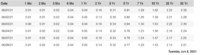# Probably easy question about Treasury debt

#### nosit

##### Junior Member
Hi!
I am not American and I am trying to understand how the interest of US Treasury debt is paid. I know their respective maturity:

T-bills: up to and including 1 year
T-notes: 2 to 10 years
T-bonds: >10 years

According to what I understood, taking the date of 08/Jun/2021:

Example T-bills:

If I buy T-bills of 6 months, then the price would be $960 and after 6 months I receive$1,000 (which means I would pay less than the face value and in the maturity date I get: $960 + 4% of$1,000 = $1000) The same kind of method I apply for all T-bills Example T-notes and T-bonds: T-note of 10 years, I would earn 1,53% every semester. The same is for all T-notes and T-bonds. ------> It seems to much interest and I am probably wrong. Is anyone able to confirm it or to explain how interest are paid for these 3 instruments?#### JeffM ##### Elite Member You basically have it. Treasury bills have maturiries measured in weeks; the longest term being 52 weeks (364 days). Nominally, no interest is paid. Bills are sold below the face value of the bill so that the interest income equals the difference between purchase price and the face value paid at maturity. Bonds and notes have maturities measured in years. They have a stated rate of annual interest. Interest payments are made semi-annually at one half the annual rate. •nosit #### nosit ##### Junior Member Thank you @JeffM ! So, my examples are also correct, right? #### JeffM ##### Elite Member No, your examples are not correct. I am guessing as to what the numbers under bills represent, an effective annual rate or a rate of discount. But in either case, I am quite confident that the numbers are percents so 0.04 means 0.04% or 0.0004, 4 basis points. Where you got your table from should clear that up. But, in today’s market, you are not buying that bill for$960, which implies an annual yield of about 8.5%.

On the assumption that 1.53 represents an annual rate, then you would get interest of $7.65 semi-annually on a note for$1000. If that 1.53 represents an annual yield, then you would get interest of \$7.62. Again, the source of the table should clarify what the number represents.